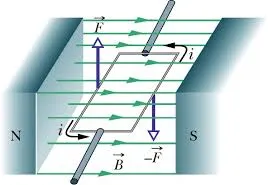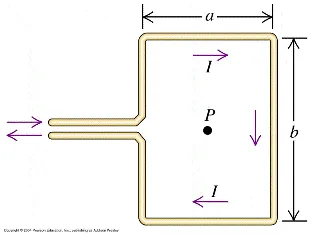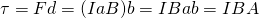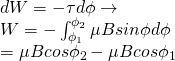# Physics - Electromagnetism - Magnetic force and torque applied on current loops (circuits)## Introduction

Hello it's a me again Drifter Programming! Today we continue with Electromagnetism to get into the Magnetic force and torque that gets exerted on current loops/circuits. To understand this post you should at least read the previous post of the series about the magnetic force on current-carrying conductors. So, without further do, let's get straight into it!

## Small recap of magnetic force

During this series 'till now, we covered what magnetic fields are and saw that they are pretty similar to electric fields, which took us to the conclusion that Electricity and Magnetism are very closely binded together. The magnetic force applied on charged particles inside of a magnetic field is proportional to the magnitude of charge of the particle, the drifting velocity and magnetic field strength (or magnetic flux density) and so follows the equation: F = qvB. Last time we wanted to find out what is true for current carrying conductors/wires in general. Current is the cause of the motion of a number of charges which got us to the conclusion that the force exerted on current carrying conductors/wires is given by the equation: F = IlxB and so proportional to the current, the length of the wire and the magnetic field strength. This last equation can of course also be written as an integral (many small straight-line conductors), which means that it can be applied on any wire. This exact equation can only be used on straight-line conductors/wires.

Something very important that you should always remember is that only the perpendicular components of velocity and current are being affected by the magnetic field, which means that charges and current flowing in parallel to the magnetic field lines are not affected by the magnetic field (total net force is zero). When in an angle we of course will have to use the corresponding component that is perpendicular and the "remaining" component that is parallel to the magnetic field will not be affected, causing the particle/current to move/flow to that direction in a helical motion.

## Magnetic force on Current Loops/Circuits

Let's consider the following rectangular current-carrying loop with side lengths a and b inside of an uniform magnetic field B:In a uniform magnetic field the net force on a current loop is zero and independent of the shape and angle of the loop. This is of course true cause the left/right and top/bottom branch forces cancel each other out. The force at the side 'a' is of course F = IaB and the force at the side 'b' is F = IbB. The current in the opposing sides is flowing in opposite directions but has the same magnitude and so do the forces, because of the right-hand rule, which means that the forces cancel each other out (F and -F).

## Magnetic moment and torque

But, does this means that the loop will not move at all? Nope, the total net force might be zero, but the total net torque (which causes rotation around an axis) isn't. From the right- hand rule we know that the bottom side causes an upward force and the top side a downward force. These two forces cause a rotation around the horizontal axis. We know that torque is calculated by: τ = Fd. Supposing the top/bottom sides have length the distance d is of course equal to the side b and so we have that the total torque is:A = ab is of course the area of the rectangular loop.

The product IA is called magnetic moment and is denoted as μ. That way for N turns the magnetic moment is given by: μ = N*IA. Another term for this quantity is magnetic dipole moment. The magnetic dipole moment is a vector and the direction can be found a right-hand rule again. The vector is perpendicular to the current loop. Magnetic moment can of course be found for any shape!

Using magnetic moment the torque can now be expressed as:

τ = μxB

The torque of course depends on the angle θ between μ and B and so has to be expressed as:

τ = μxBsinθ

The fact that current carrying loops experience a net torque in a magnetic field is the principle behind the electric motor that we will get into next time...

Something worth noting is that the potential energy can be associated with the magnetic moment using the equation:

U = -μB

The "highest" magnetic potential energy is at the point where the magnetic moment μ is opposite to the magnetic field. The difference in energy between the aligned and anti-aligned "states" is:

ΔU = 2μB

The Work produced by the magnetic torque when moving from an angle φ1 to an angle φ2 can be found by integration as:That way by rotating 180 degrees (o to π) the amount of the rotational work would be:

W = 2μB. cause cos0 = 1 and cosπ = -1.

## REFERENCES:

Mathematical equations that I had in this post where drawn using quicklatex!

### Electric fields:

Getting into Electromagnetism -> electromagnetim, electric charge, conductors, insulators, quantization

Coulomb's law with examples -> Coulomb's law, superposition principle, Coulomb constant, how to solve problems, examples

Electric fields and field lines -> Electric fields, Solving problems around Electric fields and field lines

Electric dipoles -> Electric dipole, torque, potential and field

Electric charge and field Exercises -> examples in electric charges and fields

### Electric flux:

Electric flux and Gauss's law -> Electric flux, Gauss's law

Applications of Gauss's law (part 1) -> applying Gauss's law, Gauss applications

Applications of Gauss's law (part 2) -> more Gauss applications

Electric flux exercises -> examples in electric flux and Gauss's law

### Electric potential:

Electric potential energy -> explanation of work-energy, electric potential energy

Calculating electric potentials -> more stuff about potential energy, potential, calculating potentials

Millikan's Oil Drop Experiment -> Millikan's experiment, electronvolt

Cathode ray tubes explained using electric potential -> cathode ray tube explanation

Electric potential exercises (part 1) -> applications of potential

Electric potential exercises (part 2) -> applications of potential gradient, advanced examples

### Capacitance:

Capacitors (Condensers) and Capacitance -> Capacitors, capacitance, calculating capacitance

How to solve problems around Capacitors -> combination, solving problems, simple example

Electric field energy and density -> Electric field energy, energy density

Dielectric materials -> Dielectrics, dielectric constant, permittivity and strength, how to solve problems

Electric capacitance exercises -> examples in capacitance, energy density and dielectrics

### Current, resistance and EMF:

Electric current -> Electric current, current density

Electrical resistivity and conductivity -> Electrical resistivity, conductivity, thermal coefficient of resistivity, hyperconductivity

Electric resistance -> Resistance, temperature, resistors

Electromotive Force (EMF) and Internal resistance -> Electromotive force, internal resistance

Power and Wattage of Electronic Circuits -> Power in general, power/wattage of electronic circuits

Electric current, resistance and emf exercises -> exampes in all those topics

### Direct current (DC) circuits:

Resistor Combinations -> Resistor combinations, how to solve problems

Kirchhoff's laws with applications -> Kirchhoff's laws, how to solve problems, applications

Electrical measuring instruments -> what are they?, types list, getting into some of them, an application

Electronic circuits with resistors and capacitors (R-C) -> R-C Circuit, charging, time constant, discharging, how to apply

RC circuit exercises -> examples in Kirchhoff, charging, discharging capacitor with/without internal resistance

### Magnetic field and forces:

Magnetic fields -> Magnetism, Magnetic field

Magnetic field lines and Gauss's law of Magnetism -> magnetic field lines, mono- and dipoles, Flux, Gauss's law of magnetism

The motion of charged particles inside of a magnetic field -> straight-line, spiral and helical particle motion

Applications of charged particle motion -> CERN, Cyclotrons, Synchrotrons, Cavity Magetron, Mass Spectrometry and Magnetic lens

Magnetic force applied on Current-Carrying Conductors -> magnetic force on current-carrying conductors/wires, proofs

And this is actually it for today's post and I hope that you enjoyed it!

Using the knowledge of today we will next time get into an explanation of the function of Electromotors...

C ya!

H2
H3
H4
3 columns
2 columns
1 column